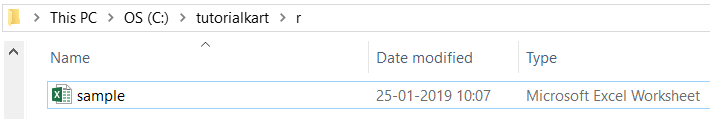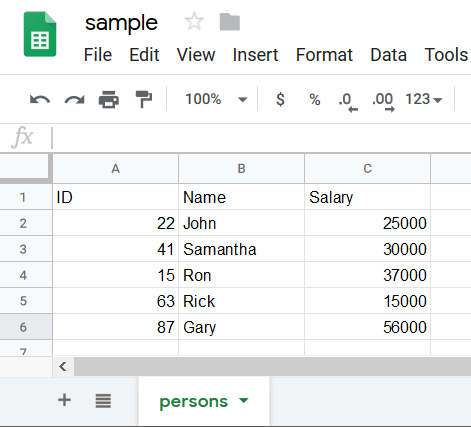In this tutorial, we will learn how to read an XLSX file in R programming.

To read Excel (XLS and XLSX) files in R, we will use the package `readxl`.

Install readxl package by running the command `install.packages("readxl")`. You should see some information echoed to the screen as shown in the below code snippet. The command installs all the dependencies.

To use the package readxl, run the following command.

Now you can start using the functions of readxl and read XLSX, XLS files.

To read an xlsx file, we can use `read_xlsx()` function or `read_excel()` function. We will learn to use these functions with examples.

The syntax of read_xlsx() function is

Except for the `path` argument (the first argument) the rest are optional.

In this example, we will consider `sample.xlsx` file containing 3 columns and five rows stored in a local drive.The contents of the XLSX file are as shown below.Now we shall run the read_xlsx() function with the path to xlsx file as argument.

Now we will go through what read_xlsx() function has read from the xlsx file.

1. It found that the size of the data is 5×3.
2. It picked the first row as Header i.e., column names for the columns.
3. It interpreted the datatypes of the columns.
1. First column <dbl> for Double.
2. Second column <chr> for Char Array.
3. Third column <dbl> for Double.

The syntax of read_excel() function is

Except for the `path` argument (the first argument) the rest are optional.

Now we shall run the read_excel() function with the path to xlsx file as argument.

The result for reading the .xlsx file with the two functions is same. The only difference is that when read_excel() is used, excel_format() is called internally by the read_excel() function to determine if the path is xls or xlsx file from the file extension.